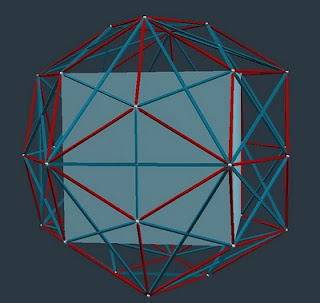## Saturday, April 10, 2010

### Radical Math and PythonFigure 1: Inscribing a Cube in a Rhombic Triacontahedron
by D. Koski

Loyal readers of these non-fiction blogs will have discovered a Russian novel's number of characters, along with numerous "mug shots" of polyhedra, repeated in many contexts.

For example, I phoned David Koski this morning to double-check on the face diagonals of the Rhombic Triacontahedron of volume 7.5 which shape, you may recall, intersects the volume 6.0 Rhombic Dodecahedron of radius 1 (or of diameter 1/2 if accounting in diameters). The 7.5 volumed Rhombic Triacontahedron has a radius of Φ/√2 compared to that of a sphere of radius 1.0.

These polyhedra are both members of our august Concentric Hierarchy of Polyhedra, a famed inner circle of polys, including the Platonic Five, that comprise our Zen Garden.

David had a ready answer: given a Cube inscribed as above has 2/5ths the Triacontahedron's volume, the 7.5 volumed RT would contain a Cube of volume 3. This is convenient, as it's the Cube volume we had already, based on two unit volume Tetrahedra intersecting each other to form its corners.

What's cool is that every time you intersect a Platonic with its dual, you get a polyhedron with rhombic faces (squares count as rhombs, and so the Cube is one of them).

When you criss-cross those rhombic faces, you divide them into four right triangles (the face diagonals of rhombs intersect at 90 degrees -- something to prove in a Euclidean geometry class). The point at the body center forms the fourth vertex of these four tri-rectangular tetrahedra per rhombic face.

In the case of the Cube and the Rhombic Dodecahedron, the fall-out from so carving the faces are Mites, or minimum tetrahedra. One gets 24 and 48 of them respectively, each weighing in with volume of 1/8. Cube: 1/8 * 24 = 3. Rhombic dodecahedron: 1/8 * 48 = 6.

In the case of the Rhombic Triacontahedron, the fall-out is the T-module shape. To be an actual T-module, you'll want your Triacontahedron to weigh in at precisely 5. This gives each of the corresponding 120 T-modules a volume of 1/24, i.e. 1/3rd that of the Mite and the same volume as the A and B modules (the Mite is comprised of 2As and 1B).

Below is David's instructive vZome as to how the Cube and Rhombic Dodecahedron are both comprised of Mites.
by D. Koski

Note that a Rhombic Triacontahedron of volume 5 would contain an inscribed cube (per Figure 1) of volume 2, i.e. 5 * 2/5 = 2. This is a good candidate for a 2-volume in the concentric hierarchy, which already has volumes 1, 3, 4, 5, 6, (Φ2 + 1) 3√2, 5 Φ2√2, 20 and 24.

Note: the above information is premised on using Tetrahedral Accounting, not widely practiced or known about as late as 2010, Dr. Arthur Loeb's brilliant essays notwithstanding.

A wily underground of Radical Math teachers, some with friends in high places, willingly circumvented the Bucky Boycott (an anti-USA PR campaign) and taught this "verboten math" on the sly -- a risky-yet-necessary business given the high stakes involved.

Python, Athena's archetypal protector (she's our goddess of wisdom, intelligence and defense) is also literally a computer language. Python proved useful for encoding such radical math as the above, keeping it alive and kicking through a dark age of rampant ignorance and tyrannical subjugation.